Inequalities

 Problem Set 1 Problem Set 2 Problem Set 3Select a problem set using the buttons above, then use your mouse or tab key to select a question. Select the button beside the correct answer for each problem. When you have answered all of the questions, ask Charlie how you did.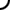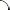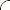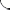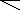Identify each statement as either true or false.   -7 > 3 true false -12 < -5 true false -4 - 5 ≤ 7 - 16 true false   For questions 4 through 6 use the diagram below. Identify each statement as either true or false.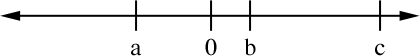b < a true false -c ≥ a true false a + b < 0 true false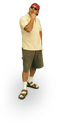Identify each statement as either true or false.   9 ≥ -1 true false -4 > -7 true false 4(5 - 3) < 72 ÷ 9 true false   For questions 4 through 6 use the diagram below. Identify each statement as either true or false.c > a true false -b > c true false c - b ≤ 0 true falseIdentify each statement as either true or false.   -5 > -6 true false 0 ≤ -4 true false 7 • 13 > 10 • 9 true false   For questions 4 through 6 use the diagram below. Identify each statement as either true or false.c ≤ b true false -a > b true false 0 < a + c true false©  2009  LarryPerezWeb Design:  Patrick Quigley